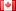# Revision history [back]

### inverse of a polynomial modulo another polynomial

Hi, I'm trying to implement the Baby Step Giant Step algorithm in the group of units of prime fields. I would like to generate the field provided one generator polynomial. But I need to calculate p^(-1) (where p is a polynomial), but can't find a function to do so. This is what I'm doing,

m = sqrt(S.modulus().degree()); gamma = S.modulus(); alpha = x^3+1;

now i need to calculate (alpha)^(-1) modulo gamma

Any help? Better ways to do the same thing?

Thanks!2 No.2 RevisionDSM5212 ●21 ●68 ●111

### inverse of a polynomial modulo another polynomial

Hi, I'm trying to implement the Baby Step Giant Step algorithm in the group of units of prime fields. I would like to generate the field provided one generator polynomial. But I need to calculate p^(-1) (where p is a polynomial), but can't find a function to do so. This is what I'm doing,

F.

F.<a> = GF(2)[];
R. R.<b> = PolynomialRing(F)
S.<x> = R.quotient(b^4+b+1)R.quotient(b^4+b+1)
m = sqrt(S.modulus().degree());
gamma = S.modulus();
alpha = x^3+1;x^3+1;


now i need to calculate (alpha)^(-1) modulo gamma

Any help? Better ways to do the same thing?

Thanks!